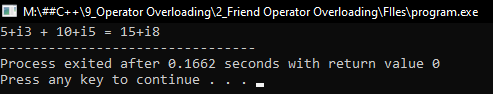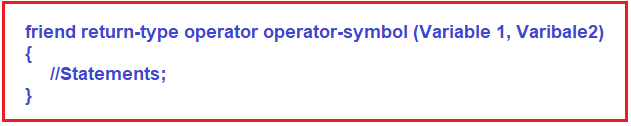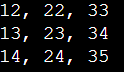In our previous article, we have already seen how to overlord unary (++, –) and binary (+) operators in C++ with Examples. There is one more method for overloading an operator in C++ that is using the friend function. Let us learn it through the same example that is using the same Complex class. The following is the sample code that we have created in our previous article.

```class Complex
{
private:
int real;
int img;
public:
Complex (int r = 0, int i = 0)
{
real = r;
img = i;
}
{
Complex temp;
temp.real = real + x.real;
temp.img = img + x.img;
return temp;
}
void Display()
{
cout << real << "+i" << img <<endl;
}
};

int main()
{
Complex C1 (3, 7);
C1.Display();
Complex C2 (5, 2);
C2.Display();
Complex C3;
C3.Display();
}

```

Suppose we want to add two complex numbers i.e. C1 and C2,

C3 = C1 + C2;

We have created add function in class Complex. At that time the idea was that either C1 will add to C2 or C2 will add to C1. But now we want somebody else to add two complex numbers.

We have also given you an example that if ‘X’ has some money and ‘Y’ also has some money and they wanted to add their money. So ‘X’ can add money or ‘Y’ can add money or they can also take help from another person i.e. friends. If their friend is adding the money then they both have to give their money to him as the parameter. Then only their friend can add the money. So, the same approach will follow for the friend function. Here we are writing the Complex class,

```class Complex{
private:
int real;
int img;
public:
friend Complex operator + (Complex C1, Complex C2);
};
```

In the above example, we have created two integer type private data members real and img. Then we overloaded the + operator with two parameters C1 and C2. We have not defined the body here. We have made it a friend by using the friend function. This is the prototype of the friend function in C++. This function will return an object of type Complex. So, this friend function will take two complex numbers as parameters and return a Complex number.

C3 = C1 + C2;

It is just like there is a function that will take C1 and C2 as parameters and add them and return the result. So, neither C1 nor C2 adding but someone else is adding. This friend function has to be written outside the class without using scope resolution. Now let us write the body of the friend function ‘operator +’ outside the class,

```Complex operator + (Complex C1, Complex C2){
Complex t;
t.real = C1.real + C2.real;
t.img = C1.img + C2.img;
return t;
}
```

This function doesn’t belong to the class but it is a friend of the Complex class. So, we don’t use any scope resolution operator. So, this is another approach to overloading operators in C++. So, operators, we can overload as a member function as well as we can overload them as friend functions. Now let us write the complete program in C++.

```#include <iostream>
using namespace std;
class Complex
{
private:
int real;
int img;
public:
Complex (int r = 0, int i = 0)
{
real = r;
img = i;
}
void Display ()
{
cout << real << "+i" << img;
}
friend Complex operator + (Complex c1, Complex c2);
};

Complex operator + (Complex c1, Complex c2)
{
Complex temp;
temp.real = c1.real + c2.real;
temp.img = c1.img + c2.img;
return temp;
}

int main ()
{
Complex C1(5, 3), C2(10, 5), C3;
C1.Display();
cout << " + ";
C2.Display();
cout << " = ";
C3 = C1 + C2;
C3.Display();
}
```
###### Output:We need to remember the following pointers while working with Operator Overloading in C++ Using Friend Function.

1. The Friend function in C++ using operator overloading offers better flexibility to the class.
2. The Friend functions are not a member of the class and hence they do not have ‘this’ pointer.
3. When we overload a unary operator, we need to pass one argument.
4. When we overload a binary operator, we need to pass two arguments.
5. The friend function in C++ can access the private data members of a class directly.
6. An overloaded operator friend could be declared in either the private or public section of a class.
7. When redefining the meaning of an operator by operator overloading the friend function, we cannot change its basic meaning. For example, we cannot redefine minus operator + to multiply two operands of a user-defined data type.
##### Syntax to use Friend Function in C++ to Overload Operators:##### Using Friend Function to Overload Unary Operator in C++:

We can also overload a unary operator in C++ by using a friend function. The overloaded ++ operator relative to the Test class using a member function is shown in the below example.

```#include <iostream>
using namespace std;
class Test
{
int a, b, c;
public:
Test()
{
a = b = c = 0;
}
Test(int i, int j, int k)
{
a = i;
b = j;
c = k;
}

// use a reference to overload the ++
friend Test operator ++ (Test & op1);
friend Test operator ++ (Test & op1, int not_used);

void Display();
};

/* Overload prefix ++ using a friend function.
This requires the use of a reference parameter. */
Test operator ++(Test & op1)
{
op1.a ++;
op1.b ++;
op1.c ++;
return op1;
}

/* Overload postfix ++ using a friend function.
This requires the use of a reference parameter. */
Test operator ++ (Test & op1, int not_used)
{
Test temp = op1;
op1.a ++;
op1.b ++;
op1.c ++;
return temp;
}

// Display a, b, c coordinates.
void Test::Display()
{
cout << a << ", ";
cout << b << ", ";
cout << c << "\n";
}

int main()
{
Test a (12, 22, 33);
a.Display();

++a;	// prefix increment
a.Display();

a++;	// postfix increment
a.Display();

return 0;
}
```
###### Output:Note: In general, you should define the member function to implement operator overloading, friend function has been introduced for a different purpose that we are going to discuss in our upcoming articles.